# User:IssaRice/Reflections on working through Tao's Analysis

Some thoughts I've had while working through Tao's Analysis I (and probably soon Analysis II).

• Notational pedantry: I like how Tao almost always introduces variables by explicitly naming their type. Even after having used$X$ to denote a subset of the reals throughout a chapter, he still begins each definition (that uses some subset of the reals) with "Let$X$ be a subset of the real line" (or similar). I had seen some other mathematicians like Tim Gowers say "Always introduce your variables to the reader before going on to talk about them", but in my experience most writers don't actually follow through on this. Vipul complains about the usual mathematical practice here.
Similarly, I like how Tao generalizes notation like that of limits to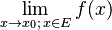$\lim_{x\to x_0;\, x\in E} f(x)$, where the "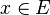$x\in E$" allows us to pass in a separate set$E$. This allows us to define e.g. left and right limits separately as instances of this general notation.
Similarly, he never writes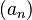$(a_n)$, which many people do (and which leaves the bound variable$n$ looking like a free variable).
• some of the notation, like n++, a--b, a//b, LIM,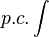$p.c.\int$, and some of the terms, like eps-close, eps-adherent, etc. are non-standard. But i like this since it tells you that you can be creative in your terms/notation. you can build things up in an idiosyncratic way, and still reach results that everybody else knows. you build everything up by hand so you know everything about how these things work.
• also, here's a note that may restore the cosmic balance: in the book, we show that$a{-}\!\!{-}b = a-b$,$a//b = a/b$, and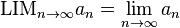$\mathrm{LIM}_{n\to\infty} a_n = \lim_{n\to\infty} a_n$. But what is the analogous result for ++? It's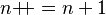$n{+}\!\!{+} = n+1$, but the notation doesn't seem to match like the others! Here's one way to think about it: if you want the increment operation to visually resemble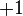$+1$, it could be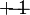${+}\!\!{-}\!\!{-}\!\!\!\!{1}$, i.e., a + and a 1 stuck together. But that actually looks just like ++ (especially in handwriting). Now all of the formal operations have a pattern to them: the formal version visually resembles the actual version (but it's "busier"/visually messier).
• Definition, example, theorem, proof: I had previously thought that I didn't like this style of writing mathematical texts, but somehow I like it in this text, so that tells me I need to introspect on this practice more, to see what specifically I like/don't like about it. It might be the case that I don't like terse/formal writing, and terse/formal writing in math textbooks tends to accompany definition, example, theorem, proof style. Informal/conversational/intuitive writing tends to be in blog posts or short articles and these tend not to have def/ex/thm/proof style. Tao does this weird thing where he adopts the def/thm/proof style but does it in a more conversational/motivated way. at least, that's one explanation i could give for why i like it.
• plain variables work just fine: Tao rarely uses bolding for variables, does not use calligraphic letters, does not use arrows over variables, etc. e.g. vectors are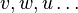$v,w,u\dots$ rather than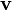$\mathbf v$ or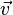$\vec v$. This does not make his writing any less clear, because he gives the type of each variable and takes care to use groups of letters (like a,b,c vs x,y,z) for separate things.
• Tao manages prerequisites by explaining even the most basic things, which is why his explanations seem clearer to a larger audience than otherwise. Eliezer used the same strategy in the Sequences.
• At each theorem, check necessity of hypotheses. Two ways of doing this: (1) remove each hypothesis individually to see that the theorem doesn't hold by coming up with counterexamples; (2) in the proof of the theorem, point out where each hypothesis is being used.
• At each definition, give both positive and negative examples. (I wish there was more of an emphasis on giving "edge cases" though.)
• Using "iff" instead of "if" in definitions.
• If a definition that introduces other variables, check the assumptions about those variables. example: why does$x_0$ need to both be in$X$ and be a limit point of$X$. (see remark 9.3.11 for another example)
• For each definition of an operation, check that it is well-defined.
• For each "superseding" definition, check consistency with old definition. examples: addition defined on reals, after we have defined addition on rationals. Infinite/arbitrary cartesian product, after we have defined finite cartesian product.
• For each definition that "clobbers up" a notation, check consistency with old definition (See inverse image for functions).
• Definitions: Disambiguate similar-seeming concepts: example of distinct vs disjoint set. strictly monotone vs monotone (one implies the other, but the converse doesn't hold).
• "(Why?)": this feels similar to a "not implemented" error in programming. it's also like the usual practice of breaking things off to lemmas, to make a proof more modular. You get to see the higher-level thoughts, rather than seeing one higher-level thought, then being forced to think through low-level details, then coming back for the next higher-level though, then going down again, ... . The latter can get tiring, so these "(why?)"s help to force the low-level details to go away. I think if this book was available as a web page, it would be cool if you could click on the "(why?)" to see the answer in pop-up window (sort of like how some websites do footnotes now).
• rolling up concepts to make them more processable: epsilon-close, epsilon-adherent, continually epsilon-close, etc. see gowers blog post as well, where he does this for the definition of limit of a sequence.
• psychologically speaking, I prefer "Proof. See Exercise n.m.p." to a textbook just mentioning a result in passing and using it, or just jumping in and using it without proof. I think I like seeing the formal statement of the result. Also, i think many books just don't even mention the proposition in the text -- they just put the whole thing in the exercises. but i like having at least the proposition in the main text, with a pointer to the exercise.

Some things I sort of didn't like:

• when defining the integers, rationals, and reals, he uses the equality symbol rather than the equivalence symbol, which makes checking that operations are well-defined a bit confusing; see post by gowers on gunctions.
• hints too close to the exercise statement.
• excessive use of numbers to refer to past results. this was actually good for exercise hints but not good for other things.
• I sort of find amusing that the errata for the text is so long. Is this a fact about mathematics in general? about analysis in particular? about Tao (e.g. perhaps the way he writes in LaTeX)? But none of the errors seem very "serious" (in the sense that they can be patched up quickly/locally); this seems like an instance of the phenomenon discussed in https://www.gwern.net/The-Existential-Risk-of-Mathematical-Error
• i wish there was a solutions manual for the text. EDIT: i felt this so strongly that I decided to start writing it myself: https://taoanalysis.wordpress.com/
• for definitions that he states using his idiosyncratic terminology, i wish he gave the equivalent (more standard) way of phrasing it as well. (he does this for a while, but gives up for e.g. definition 9.9.2)
• there are no pictures in the text. in fact, at several points during the text, Tao remarks how wonderful it is that we can do everything analytically without relying on geometry. The best we get is the piston analogy for sup/limsup.
• i wish he gave more intuition/ways why a result is obvious: some examples i can think of are (1) his proof of the Cauchy condensation test, where the result is obvious if you write out the series, whereas Tao dives straight into a proof; and (2) when he proves proposition 4.3.7(h) just using algebra, whereas it's obvious if you draw a picture. (3) his proof of the alternating series test looks just like symbol shuffling to me; i had to attempt this proof over multiple days to see for myself why it made sense. (4) the hint for proving the mean value theorem will get you the proof, but it doesn't really tell you why it should work. some books use the function that measures the difference between the given function f and the line segment joining the points (a, f(a)), (b, f(b)). i think this is better, but still not completely clear (it's also not clear how i would have come up with this).
• i wish he did more of the gowers thing of writing out wrong definitions and seeing why they are wrong. see also vipul's pages on error-spotting exercises.